#### Numerical question is a type of question where you enter the answer numerically. It looks very similar to the short answer question, but you can set the allowable error range and unit of the correct answer when you set the answer. *Set the allowable error range of the correct answer: If there is a question with the allowable error = 5 and the correct answer value = 100, 95 to 105 will be regarded as the correct answer. *Set the unit: if there is a question with the correct answer value = 1000 and the unit = km/m/cm, 1km, 1000m and 100000cm are regarded as the correct answer.

Refer to:
10-01-20.How to use quiz sample questions in your course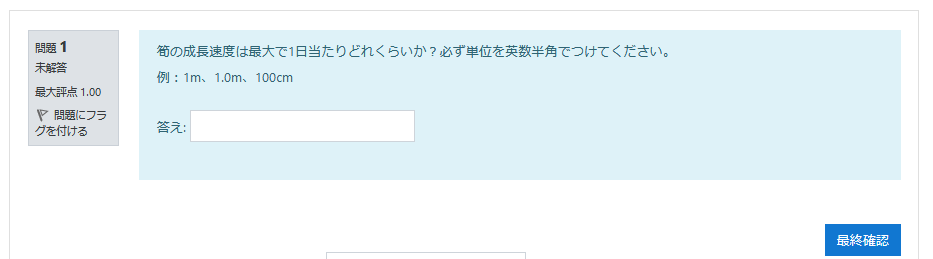1. Choose [Numerical] question and click [Add].2. Enter “Question name” and “Question text.” Enter “Default mark.”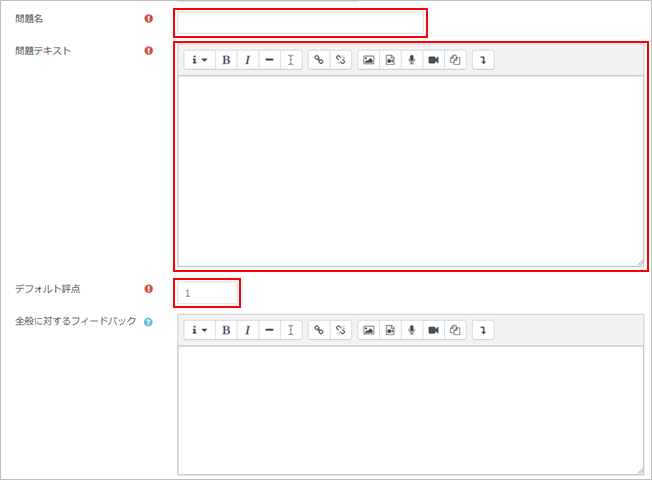memo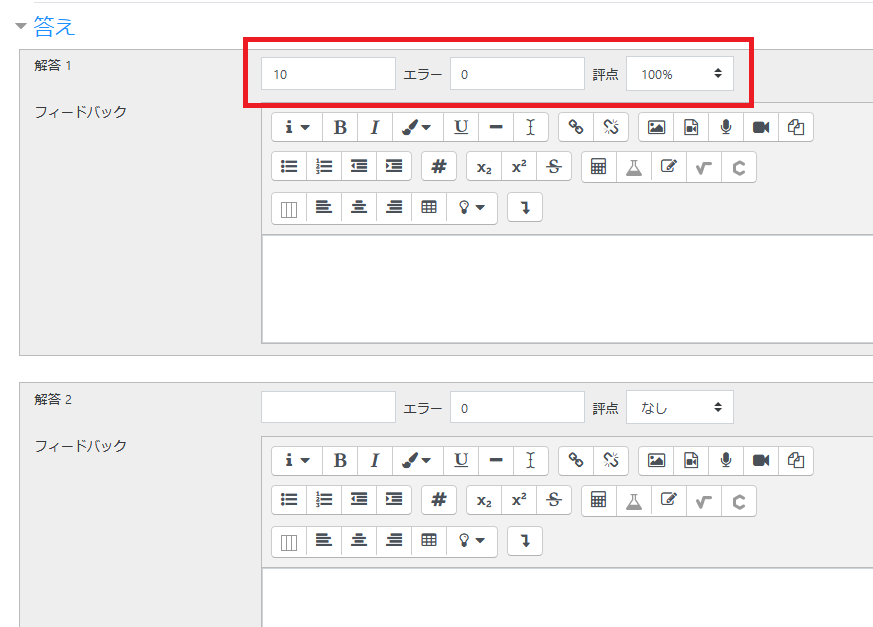4. Set “Units” if needed.
memo
If “m” is set as a unit for the question such as calculation of length, the answer would be 100 or 100m if the correct value = 100.
You can also set the multiplier for each unit. If you set “unit: km, multiplier: 0.001,” “unit: cm, multiplier: 100,” then “100,” “100m,” “0.001km,” “100cm” will be the correct answer.“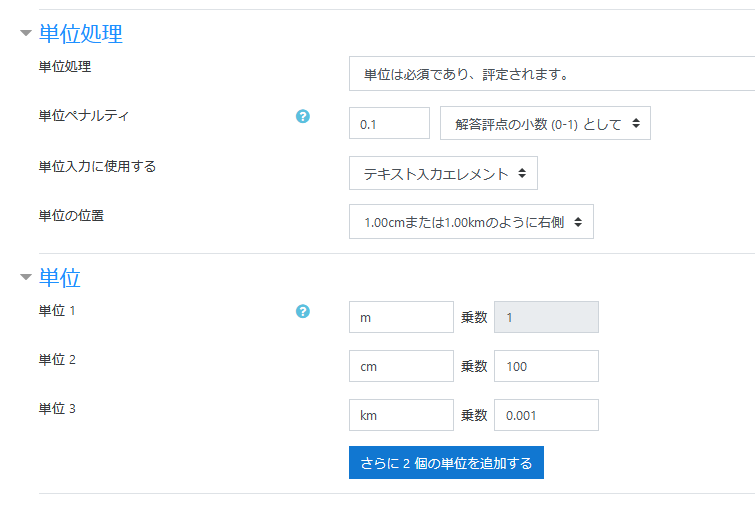5. Click [Save changes] and the numerical question will be added.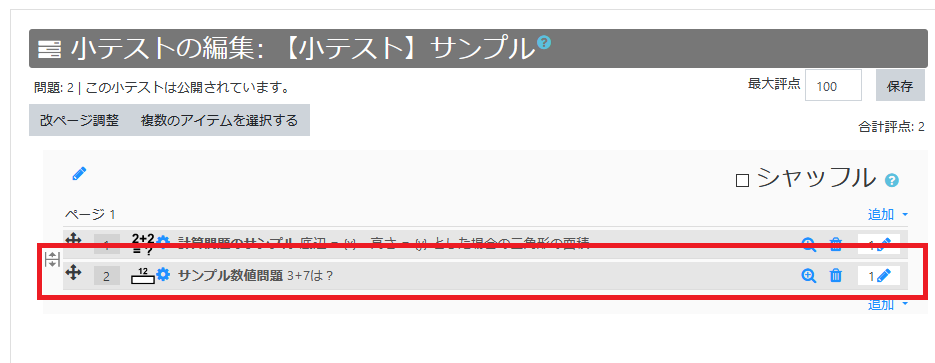6. Check the question on the preview display.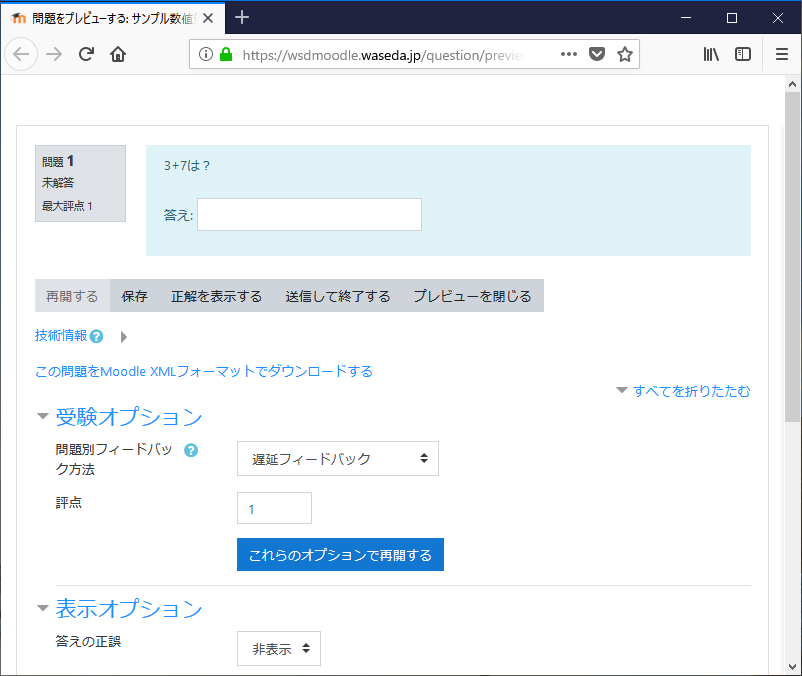Last Updated on 2021-03-30